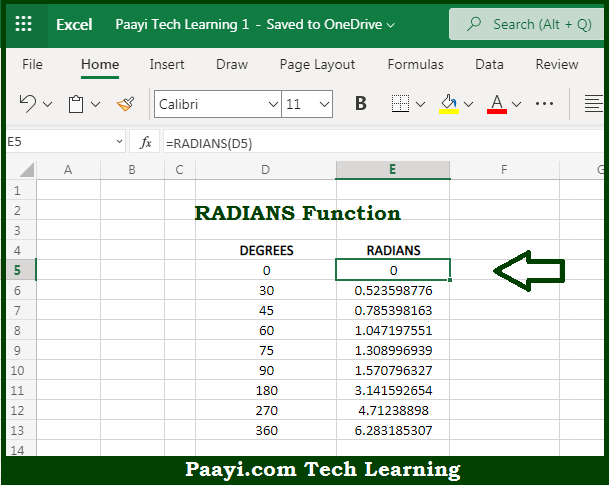# Learn How to Use Microsoft Excel RADIANS Function

Written by | 0 Comments | 571 Views

In this article, you will learn how to use the Microsoft Excel RADIANS function and its prime function in Microsoft Excel. You will also get to know the Microsoft Excel RADIANS function return value and syntax with the help of some examples.

The main purpose of the Microsoft Excel RADIANS function is to convert the degrees into radians. That implies, with the help of the RADIANS function you can able to convert the angles provided in degrees to RADIANS. So, with the help of the RADIANS function, you can able to convert the degrees into the radians.

The return value will be the radians.

Where the arguments:

• angle: This is the angle provided in degrees, which you want to convert in radians.

## How to Use Microsoft Excel RADIANS Function?So we know that Microsoft Excel RADIANS function you can able to convert the degrees into radians. That implies, with the help of the RADIANS function you can able to convert the angles provided in degrees to RADIANS. So, with the help of the RADIANS function, you can able to convert the degrees into the radians.